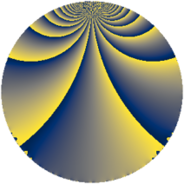# Properties

 Label 165.2.sLevel $165$ Weight $2$ Character orbit 165.s Rep. character $\chi_{165}(4,\cdot)$ Character field $\Q(\zeta_{10})$ Dimension $48$ Newform subspaces $1$ Sturm bound $48$ Trace bound $0$

# Related objects

## Defining parameters

 Level: $$N$$ $$=$$ $$165 = 3 \cdot 5 \cdot 11$$ Weight: $$k$$ $$=$$ $$2$$ Character orbit: $$[\chi]$$ $$=$$ 165.s (of order $$10$$ and degree $$4$$) Character conductor: $$\operatorname{cond}(\chi)$$ $$=$$ $$55$$ Character field: $$\Q(\zeta_{10})$$ Newform subspaces: $$1$$ Sturm bound: $$48$$ Trace bound: $$0$$

## Dimensions

The following table gives the dimensions of various subspaces of $$M_{2}(165, [\chi])$$.

Total New Old
Modular forms 112 48 64
Cusp forms 80 48 32
Eisenstein series 32 0 32

## Trace form

 $$48q + 12q^{4} - 4q^{5} + 4q^{6} + 12q^{9} + O(q^{10})$$ $$48q + 12q^{4} - 4q^{5} + 4q^{6} + 12q^{9} - 12q^{10} - 4q^{14} + 10q^{15} - 44q^{16} - 16q^{19} + 46q^{20} - 32q^{21} - 12q^{24} + 14q^{25} - 76q^{26} + 4q^{30} - 20q^{31} - 24q^{34} - 40q^{35} - 12q^{36} - 8q^{39} - 72q^{40} + 60q^{41} - 48q^{44} + 4q^{45} + 108q^{46} - 28q^{49} - 38q^{50} + 28q^{51} + 16q^{54} - 20q^{55} + 24q^{56} + 60q^{59} + 48q^{60} + 40q^{61} + 64q^{64} + 20q^{65} + 12q^{66} + 20q^{69} + 86q^{70} - 32q^{71} - 32q^{74} - 40q^{75} - 136q^{76} - 52q^{79} + 42q^{80} - 12q^{81} - 70q^{85} - 104q^{86} + 40q^{89} - 8q^{90} - 40q^{91} + 72q^{94} - 2q^{95} + 28q^{96} + O(q^{100})$$

## Decomposition of $$S_{2}^{\mathrm{new}}(165, [\chi])$$ into newform subspaces

Label Dim. $$A$$ Field CM Traces $q$-expansion
$$a_2$$ $$a_3$$ $$a_5$$ $$a_7$$
165.2.s.a $$48$$ $$1.318$$ None $$0$$ $$0$$ $$-4$$ $$0$$

## Decomposition of $$S_{2}^{\mathrm{old}}(165, [\chi])$$ into lower level spaces

$$S_{2}^{\mathrm{old}}(165, [\chi]) \cong$$ $$S_{2}^{\mathrm{new}}(55, [\chi])$$$$^{\oplus 2}$$